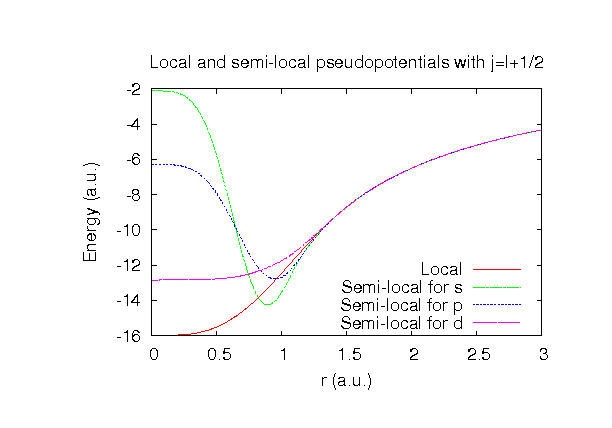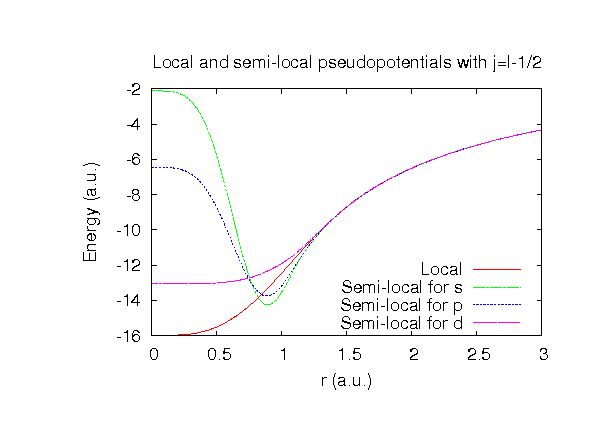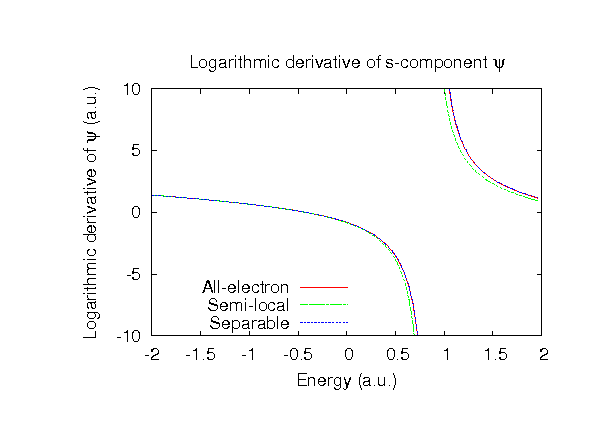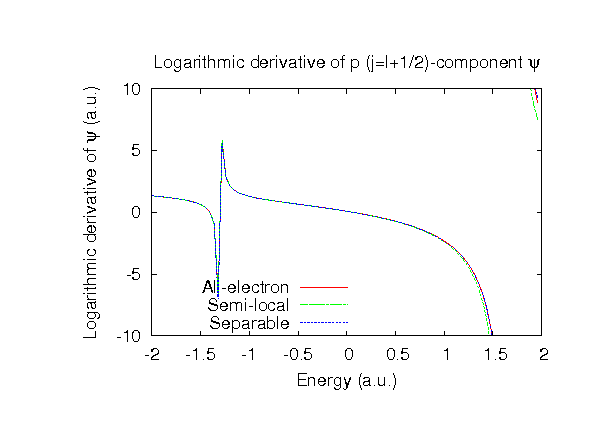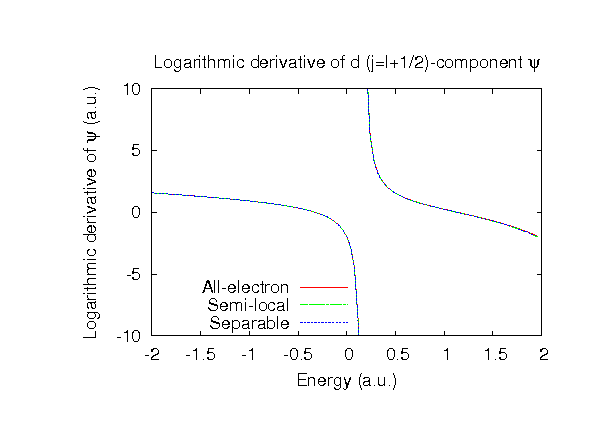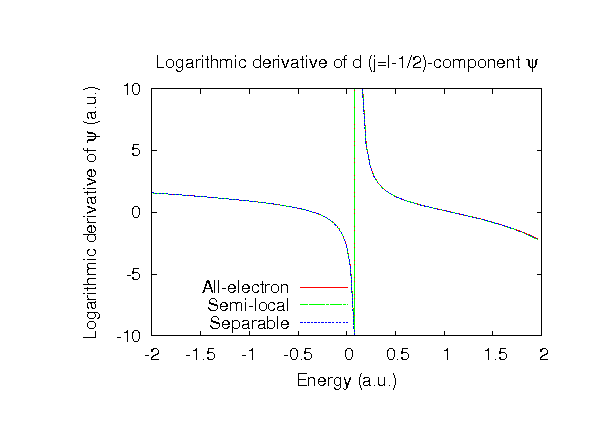### Fully relativistic pseudopotentials

Fully relativistic pseudopotentials generated by the MBK (PRB 47, 6728 (1993)) scheme within LDA (CA13) and GGA (PBE13) which contain a partial core correction and fully relativistic effects including spin-orbit coupling.

### Pseudo-atomic orbitals

The number below the symbol means a cutoff radius (a.u.) of the confinement potential. These file includes fifteen radial parts for each angular momentum quantum number l (=0,1,2,3). The basis functions were generated by variationally optimizing the corresponding primitive basis functions in the single atom, the BCC bulk, and and the dimer molecule. The input files used for the orbital optimization can be found at Ta_opt.dat and Tabcc_opt.dat. Since Ta_CA13.vps and Ta_PBE13.vps include the 5s, 5p, 5d, and 6s states (13 electrons) as the valence states, the minimal basis set is Ta*.*-s2p1d1. Our recommendation for the choice of cutoff radius of basis functions is that Ta7.0.pao is enough for bulks, but Ta9.0.pao or Ta11.0.pao is preferable for molecular systems.

### Benchmark calculations by the PBE13 pseudopotential with the various basis functions

(1) Calculation of the total energy as a function of lattice constant in the bcc structure, where the total energy is plotted relative to the minimum energy for each case. a0 and B0 are the equilibrium lattice constant and bulk modulus obtained by fitting to the Murnaghan equation of state. The difference between Ta7.0-s3p2d2f1 and Ta7.0-s3p3d3f2 in the total energy at the minimum point is 0.0043 eV/atom. An input file used for the OpenMX calculations can be found at Tabcc-EvsV.dat. For comparison the result by the Wien2k code is also shown, where the calculation was performed by default setting in the Ver. 9.1 of Wien2k except for the use of RMT x KMAX of 11.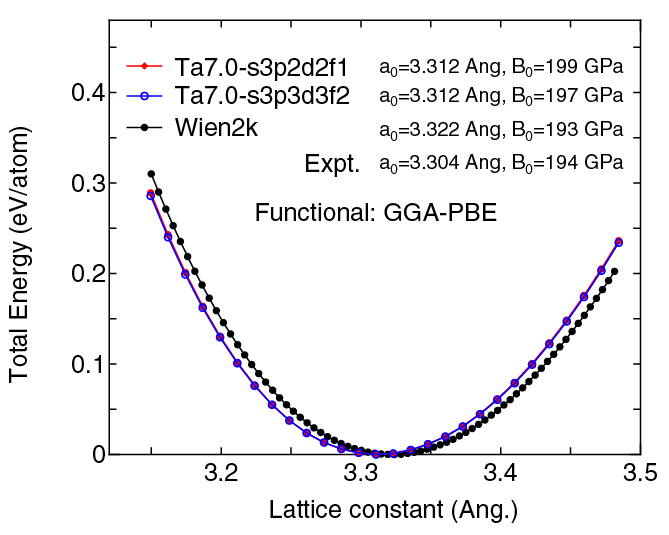(2) Calculations of the band dispersion in the bcc structure, where the non-spin polarized collinear calculation with the lattice constant of 3.300 Ang. was performed using Ta_PBE13.vps and Ta7.0-s3p2d2f1, and the origin of the energy is taken to be the Fermi level. The input file used for the OpenMX calculations can be found at Tabcc-Band.dat. For comparison the result by the Wien2k code is also shown, where the calculation was performed by default setting in the Ver. 9.1 of Wien2k except for the use of RMT x KMAX of 12.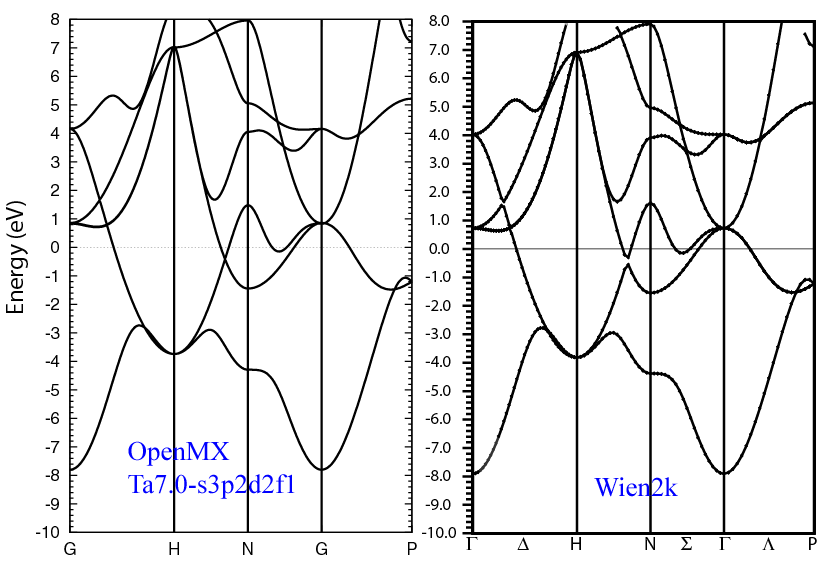### Supplementary information for the GGA (PBE13) pseudopotential